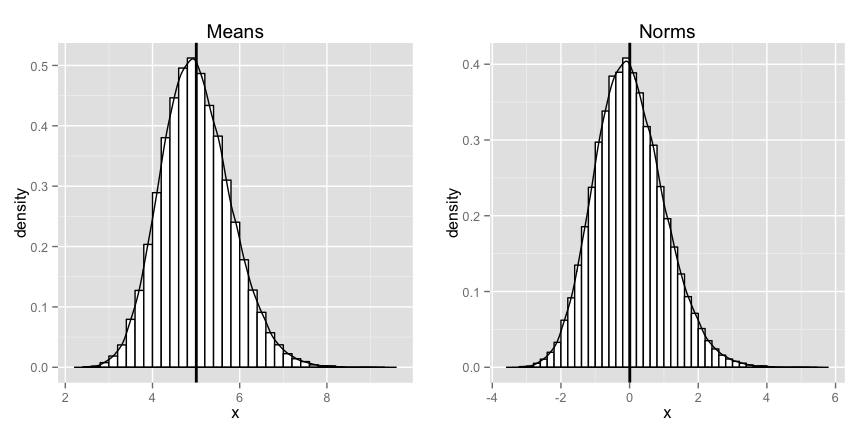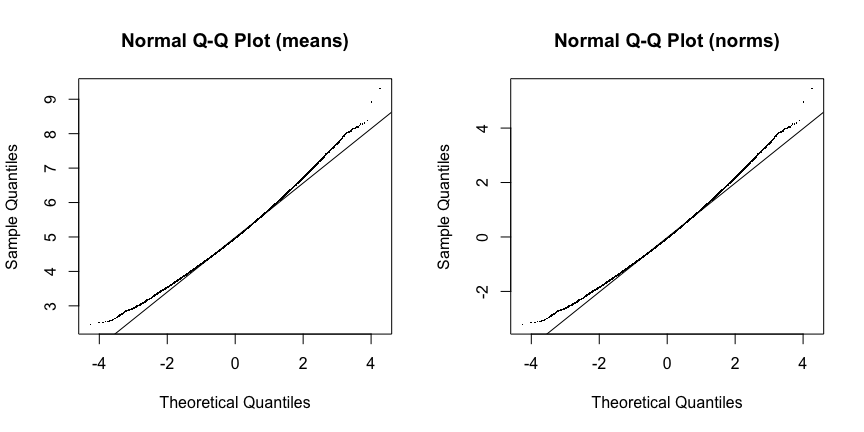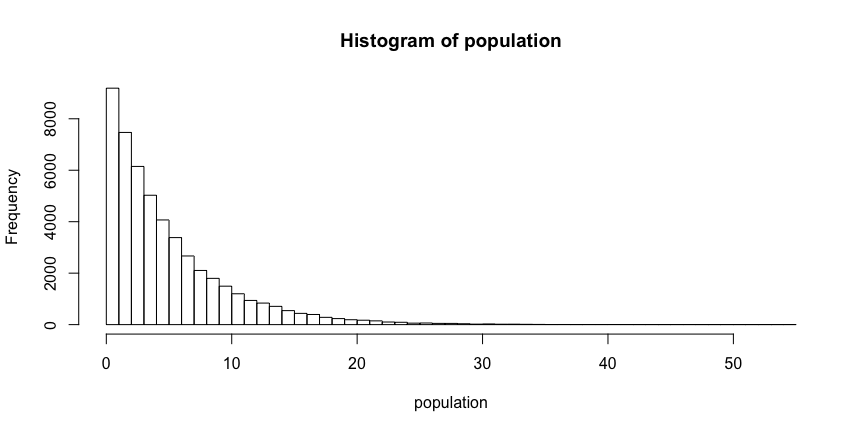The Introduction

From wikipedia:

In probability theory, the central limit theorem (CLT) states that, given certain conditions, the arithmetic mean of a sufficiently large number of iterates of independent random variables, each with a well-defined expected value and well-defined variance, will be approximately normally distributed, regardless of the underlying distribution.

In other words the distribution of sample statistics is nearly normal, centered at the population mean, and with a standard deviation equal to the population standard deviation divided by square root of the sample size. We will investigate the CLT through a simulation.

The Simulation

Our simulation will draw 40 samples from an exponentially distributed population. It will construct both a set of means of these samples, and a normalized set of means of these samples. We will analyze these datasets to see if they are consistent with what the CLT predicts. First we define the population parameters of the underlying distribution:

lambda    <- 0.2;
mean      <- 1 / lambda;
var       <- 1 / lambda^2;
sd        <- 1 / lambda;

Next we set up the parameters of the simulation, our normalization function, and generate our simulated samples:

n         <- 40;
count     <- 50000;

mean_s    <- mean;
var_s     <- var / n;
sd_s      <- sd / sqrt(n);

normalize <- function(x) (mean(x) - mean_s) / sd_s;

samples   <- matrix(rexp(count * n, rate = lambda), nrow = count, ncol = n);
means     <- data.frame(x = apply(samples, 1, mean));
norms     <- data.frame(x = apply(samples, 1, normalize));

The Analysis

Before we visualize the results of the simulation, we summarize the results - we compare the mean and the standard deviation of the simulation with the theoretical mean and standard deviation:

values            <- c(mean(means$x), var(means$x), sd(means$x), mean_s, var_s, sd_s); compare <- matrix(values, nrow = 3, ncol = 2, byrow = FALSE); colnames(compare) <- c('simulated', 'theoretical'); rownames(compare) <- c('mean', 'variance', 'standard deviation'); compare; ## simulated theoretical ## mean 5.0045121 5.0000000 ## variance 0.6330259 0.6250000 ## standard deviation 0.7956292 0.7905694 summary(means); ## x ## Min. :2.455 ## 1st Qu.:4.449 ## Median :4.962 ## Mean :5.005 ## 3rd Qu.:5.517 ## Max. :9.321 summary(norms); ## x ## Min. :-3.219743 ## 1st Qu.:-0.696393 ## Median :-0.048062 ## Mean : 0.005707 ## 3rd Qu.: 0.653416 ## Max. : 5.465812 The simulated statistics agree with the throretical statistics to two or more decimal places. Next we plot the results of the simulation - the means and the normed means - side by side. We use a histogram, and overlay a density curve for each distribution to emphasize the shape of the curve: plot1 <- ggplot(data = means, aes(x = x)); plot1 <- plot1 + geom_histogram(aes(y = ..density..), binwidth = 0.2, color = 'black', fill = 'white'); plot1 <- plot1 + geom_density(alpha = 0.2); plot1 <- plot1 + geom_vline(aes(xintercept = mean(means$x)),
color = 'black', size = 1);
plot1 <- plot1 + ggtitle("Means");

plot2 <- ggplot(data = norms, aes(x = x));
plot2 <- plot2 + geom_histogram(aes(y = ..density..),
binwidth = 0.2, color = 'black', fill = 'white');
plot2 <- plot2 + geom_density(alpha = 0.2);
plot2 <- plot2 + geom_vline(aes(xintercept = mean(norms$x)), color = 'black', size = 1); plot2 <- plot2 + ggtitle("Norms"); multiplot(plot1, plot2, cols = 2);Visually alone the plots both look normal, as we would expect from the CLT. Next we generate a qqplot of both datasets to see how they differ from the standard normal: par(mfrow = c(1, 2)); qqnorm(means$x, pch = '.', main = "Normal Q-Q Plot (means)");
qqline(means$x); qqnorm(norms$x, pch = '.', main = "Normal Q-Q Plot (norms)");
qqline(norms\$x);The datasets agree well with the standard normal, deviating slightly in the tails - which is what we might expect (the sample sizes are significantly smaller than the population, and hence we would expect the tails to differ). For reference, a plot of the original exponential distribution is presented below:

population <- rexp(50000, rate = lambda);
hist(population, breaks = 60);As you can see it is right skewed, and does not resemble the sampling distributions above.